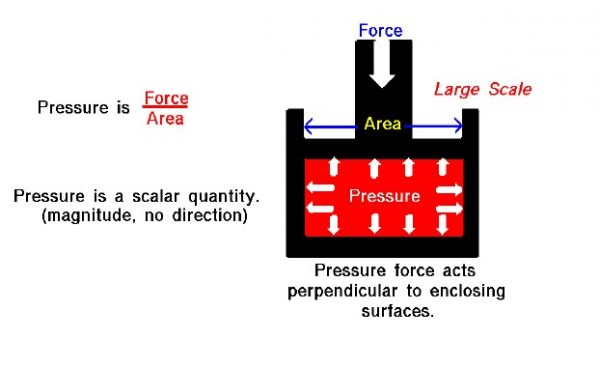# Air PressureAn important property of any gas is its pressure. We have some experience with air pressure that we don’t have with properties like viscosity and compressibility. We’ve heard meteorologists give the daily value of the barometric pressure of the atmosphere (29.8 inches of mercury, for example). And most of us have blown up a balloon or used a pump to inflate a bicycle tire or a basketball.

## Definition of Pressure

There are two ways to look at pressure: (1) the small scale action of individual air molecules or (2) the large scale action of a large number of molecules. The small scale action is described by the kinetic theory of gases and we have no evidence that the Wright brothers were familiar with this theory when they designed the aircraft and engine.
The most important result from the small scale view of pressure is that the pressure in a gas is related to the momentum of the individual moving gas molecules. Momentum is mass times velocity. So the higher the velocity of the molecules of a gas, the greater the pressure. The temperature of a gas depends on the average kinetic energy (mass times velocity squared) of the molecules of the gas, so the temperature and pressure of a gas are related to each other through an equation of state. Considering the large scale, pressure P is defined to be the force F acting on an area A divided by the area that it acts on.

## Scalar Quantity

Let us look at a gas that does not appear to move or flow. Actually, while the gas does not appear to move, the individual molecules of the gas (which we cannot see) are in constant random motion. Because we are dealing with a large number of molecules (nearly infinite) and because the motion of the individual molecules is random in every direction, we do not detect any motion. If we enclose the gas within a container, we will detect a pressure in the gas from the molecules colliding with the walls of our container. We can put the walls of our container anywhere inside the gas, and the force per area (the pressure) will be the same. (If the gas as a whole were moving, the measured pressure would be different in the direction of the motion, as described by Bernoulli’s equation.) We could shrink the size of our “container” down to an infinitely small point, and the pressure would have a single value at that point. Therefore, pressure is a scalar quantity, not a vector quantity like velocity that has a specified direction associated with it. Pressure has a magnitude but no direction associated with it. Pressure acts in all directions at a point inside a gas. At the surface of a gas, the pressure force acts perpendicular to the surface.

## Magnitude of Pressure Force

The magnitude of the pressure force is equal to the pressure (force/area) times the surface area, and the direction is perpendicular to the surface. In the figure, we have a gas (in red) that is confined in a box. A force is applied to the top of the box. The pressure force within the box opposes the applied force. And the pressure equals the external force divided by the area of the top of the box. Inside the gas, the pressure acts in all directions. So the pressure would also push on the bottom of the box and on the sides. This is different from simple solid mechanics. If the red gas were a solid, there would be no forces applied to the sides of the box; the applied force would be simply transmitted to the bottom. But in a gas, because the molecules are free to move about and collide with one another, a force applied in the vertical direction causes forces in the horizontal direction.

Provide feedback